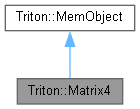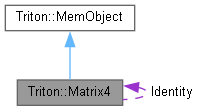Triton Visual simulation library for ocean rendering.
Triton::Matrix4 Class Reference

An implementation of a 4x4 matrix and some simple operations on it. More...

#include <Matrix4.h>

Inheritance diagram for Triton::Matrix4:[legend]
Collaboration diagram for Triton::Matrix4:[legend]

Public Member Functions

Matrix4 ()
Default constructor; initializes the matrix to an identity transform. More...

Matrix4 (double e11, double e12, double e13, double e14, double e21, double e22, double e23, double e24, double e31, double e32, double e33, double e34, double e41, double e42, double e43, double e44)
This constructor allows you to initialize the matrix as you please. More...

Matrix4 (const double *m)
Initializes the matrix from an array of 16 double-precision values (row-major). More...

~Matrix4 ()
Destructor. More...

double TRITONAPI operator() (int x, int y) const
Retrieve a specific matrix element.

void TRITONAPI ToFloatArray (float val) const
Populates a static array of 16 floats with the contents of the matrix. More...

Matrix4 TRITONAPI operator * (const Matrix4 &mat) const
Multiplies two matrices together. More...

Vector4 TRITONAPI operator * (const Vector4 &vec) const
Transform a point by the matrix. More...

Vector3 TRITONAPI operator * (const Vector3 &vec) const
Transform a point by the matrix. More...

void TRITONAPI Transpose ()
Transposes the matrix in-place. More...

Matrix4 TRITONAPI InverseCramers (double epsilon=1E-12) const
Computes the inverse of the matrix using Cramer's rule. More...

double *TRITONAPI GetRow (int row) const
Retrieves a pointer into the requested row of the matrix. More...

Public Attributes

double elem 
Data members are public for convenience.

Friends

Vector4 TRITONAPI operator * (const Vector4 &vec, const Matrix4 &mat)
Multiplies a 1x3 vector by a matrix, yielding a 1x3 vector. More...

Detailed Description

An implementation of a 4x4 matrix and some simple operations on it.

◆ Matrix4() [1/3]

 Triton::Matrix4::Matrix4 ( )
inline

Default constructor; initializes the matrix to an identity transform.

◆ Matrix4() [2/3]

 Triton::Matrix4::Matrix4 ( double e11, double e12, double e13, double e14, double e21, double e22, double e23, double e24, double e31, double e32, double e33, double e34, double e41, double e42, double e43, double e44 )
inline

This constructor allows you to initialize the matrix as you please.

◆ Matrix4() [3/3]

 Triton::Matrix4::Matrix4 ( const double * m )
inline

Initializes the matrix from an array of 16 double-precision values (row-major).

◆ ~Matrix4()

 Triton::Matrix4::~Matrix4 ( )
inline

Destructor.

◆ GetRow()

 double* TRITONAPI Triton::Matrix4::GetRow ( int row ) const
inline

Retrieves a pointer into the requested row of the matrix.

◆ InverseCramers()

 Matrix4 TRITONAPI Triton::Matrix4::InverseCramers ( double epsilon = 1E-12 ) const
inline

Computes the inverse of the matrix using Cramer's rule.

◆ operator *() [1/3]

 Matrix4 TRITONAPI Triton::Matrix4::operator * ( const Matrix4 & mat ) const

Multiplies two matrices together.

◆ operator *() [2/3]

 Vector4 TRITONAPI Triton::Matrix4::operator * ( const Vector4 & vec ) const

Transform a point by the matrix.

◆ operator *() [3/3]

 Vector3 TRITONAPI Triton::Matrix4::operator * ( const Vector3 & vec ) const

Transform a point by the matrix.

◆ ToFloatArray()

 void TRITONAPI Triton::Matrix4::ToFloatArray ( float val ) const
inline

Populates a static array of 16 floats with the contents of the matrix.

◆ Transpose()

 void TRITONAPI Triton::Matrix4::Transpose ( )
inline

Transposes the matrix in-place.

◆ operator *

 Vector4 TRITONAPI operator * ( const Vector4 & vec, const Matrix4 & mat )
friend

Multiplies a 1x3 vector by a matrix, yielding a 1x3 vector.

The documentation for this class was generated from the following file: